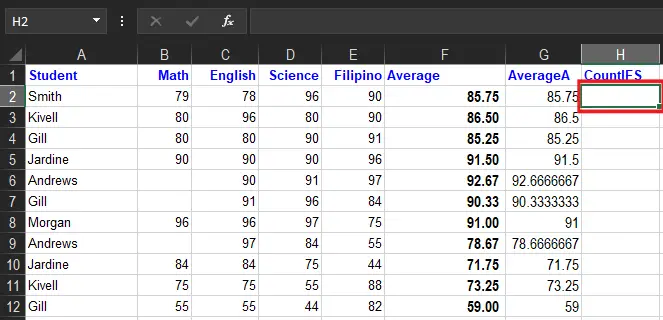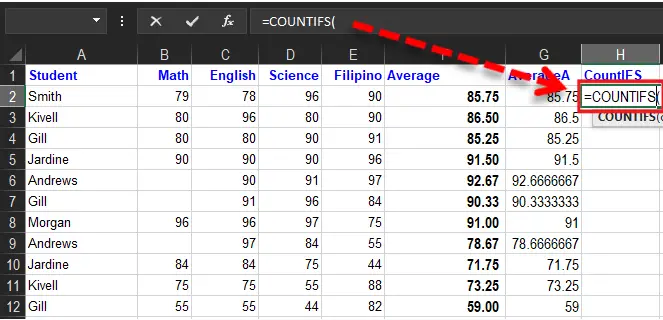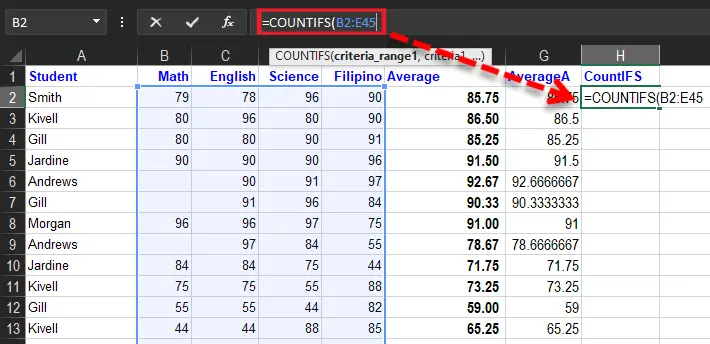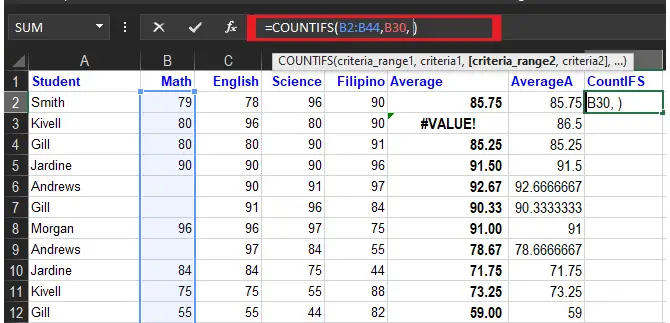# How To Use The Countifs Function In Excel In Simple Way

In this article, we will learn how to use the countifs function of excel and its description, examples, and figures to better understand it.

Prior to this function which is a premade function of Count in Excel. Hence, the plural counterpart of Excel COUNTIF function which was discovered is simple but helpful in calculations specifically in a worksheet.

## What is COUNTIFS function in Excel?

The Excel countifs is under the category of Excel statistical Functions, which counts the number of cells that meets the criteria or multiple criteria specifically, either on the same or different page.

Furthermore, the COUNTIF function helps in doing brief analyses, such as in doing a grading system. In some instances, we are given the work to do complete grades for a student, their dates of the deadline, and a list of requirements of their tasks.

The difference between COUNTIF and COUNTIFS is that COUNTIF formula is designed for counting cells with a single condition in one range, whereas COUNTIFS can evaluate different criteria in the same or different ranges.

Additionally, It applies to Excel for Office 365, Excel 2019, Excel 2016, Excel 2013, Excel 2011 for Mac, Excel 2010, Excel 2007

### Syntax

`COUNTIFS(criteria_range1, criteria1, [criteria_range2, criteria2]…)`

### Arguments

• `criteria_range1`: This is required. The first range in which evaluates associated criteria.
• `criteria1`: This is required. The criteria in the form of a number, expression, cell reference, or text define which cells will be counted. For example, criteria can be expressed as 32, “>32”, B4, “apples”, or “32”.
• `criteria_range2, criteria2, ...` This is optional. Additional ranges and their associated criteria. Up to 127 range/criteria pairs are allowed.

### Return Value

The number of times criteria are met.

## Criteria

The tough part of utilizing the COUNTIFS function is its syntax, which is a little bit complex to apply criteria. Its logical criteria are divided into two parts which are the range and criteria.

As a result, the technique of this is you need to separate the range and criteria whereas logical operators placed in the condition are enclosed in the double quote (“”).

See the example below for the logic of the syntax.

The conditions are referred to as `critera1, criteria2, ..` and so on, which can check things like:

• > If a number is greater than another number.
• < If a number is lesser than another number.
• = If a number or text is equal to something.

The `criteria_range1``criteria_range2`, and so on, are the ranges where the function checks for the conditions.

## How to Use the COUNTIFS Function in Excel

Time needed: 3 minutes.

Example COUNTIFS function, step by step:

1. Select the blank cell `H3`2. Type `=COUNTIFS`3. Double-click the COUNTIFS command.

4. Specify the range for the type range B2:B44(the Type 1 values)5. Type comma (`,`).

6. Specify the criteria.7. Type comma (`,`).

8. Specify the range for the second condition `A2:A44`.

9. Type comma (`,`).

10. Specify the criteria (the cell A30, which has the value “Jones”)

11. Hit enter to see the result.### Dates

The best way to use COUNTIFS using dates is to direct to the valid date of another cell or reference. This will count cells from B2:B44 which contains greater than date, you may use this formula:

• `=COUNTIFS(B2:A10, ">"&B2)`` count dates greater than b2`.

As you noticed the less than operator is concatenated to the date present in B2, however there are no quotes in a cell reference.

Meanwhile , there is the safest way to use date as criteria in COUNTIFS is the DATE function in excel. It ensures that Excel will read as a date. For instance, observe the formula below.

• `=COUNTIFS(A2:A44,"<"&DATE(2022,12,3)) it means dates less than December 1, 2022`

### Wildcards

The countifs function also uses wildcards as criteria. So wildcard characters are the question mark (?), asterisks (*), or tilde (~).

So using an asterisk (*) matches zero or more characters of any kind, and a question mark (?) matches any single character. Meanwhile, the tilde (~) is an escape character to allow you to find literal wildcards. For example, to count cells in A1:A44 that contain the text “Smith” anywhere, you can use a formula like this:

`=COUNTIFS(A1:A44,"*Smith*") ``It count cells that contain "Smith"`.

### Reminders about the COUNTIFS Function:

1. COUNTIFS is functional from MS Excel 2000.
2. #VALUE! erro means:
• The length is not equall to the given criteria_range arrays.
• The text strings that are supplied criteria in a arguments are greater than 255 characters long.
3. The additional range needs to include the same number of rows and columns along with the Criteria_range1 argument although it is necessary that they aren’t adjacent to each other.

### Limitations

Here are the limtaions of countifs function you should be aware of:

• The Conditions in countif is accompanied by the AND logic. In short it should be true in all conditions in order to count the cell.
• COUNTIFS is not case-sensitive. To count values based on the case sensitive function used a formul SUMPRODUCT Excel exact functiobn.
• The Countis function alter values appears in a range argument before appying criteria. It requires the actual range for all the ange argument, just don’t use it as an array.

The easiest way to work around the limitations is using the SUMPRODUCT function. Alternatively, the BYROW and BYCOL of the newest version of Excel works with it.

## Summary

In summary, we learn how to use the COUNTIFS Function in Excel In a simple way. It is a bit complex but it best in compplying the task especially, counting on set of list with a specific criteria. If you will master it is helpful in working your every task aswell as a skill that you could obtain in learning it.